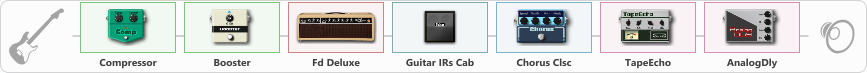# hasuhn

Discussion in 'ToneLib-GFX presets' started by selcuk, Jul 22, 2023.

1. hasuhn

Preset name: Sweet Fender Deluxe Chorus

Effects chain:Effect: "Compressor" (Dynamics / Filter), active - "yes"
{
"Sense" = 44
"Level" = 50
}

Effect: "Booster" (Dynamics / Filter), active - "yes"
{
"Gain" = 31
}

Effect: "Fd Deluxe" (Amp simulators), active - "yes"
{
"Gain" = 50
"Bass" = 51
"Middle" = 56
"Treble" = 61
"Presence" = 50
"Master" = 73
"Level (dB)" = 3
}

Effect: "Guitar IRs Cab" (Cabinets), active - "yes"
{
"Model" = Fender Deluxe Rev (1x12")
"Mic Position" = Center
"Mic Distance" = Near
"Low Cut (Hz)" = 60
"Hi Cut (kHz)" = 20.0
"Mix" = 100
"Level (dB)" = 0
}

Effect: "Chorus Clsc" (Modulation / Sfx), active - "yes"
{
"Speed" = 3.1
"Depth" = 36
"Center" = 1.0
"Mode" = Stereo
}

Effect: "TapeEcho" (Delay), active - "yes"
{
"Time" = 316
"Feedback" = 32
"Tone" = 65
"LoDamp" = 19
"Mix" = 65
}

Effect: "AnalogDly" (Delay), active - "yes"
{
"Time" = 359
"Feedback" = 34
"Tone" = 65
"Mix" = 65
}

Note: You will need to download and install the ToneLib-GFX software to use the preset.

File size:
675 bytes
Views:
1,410